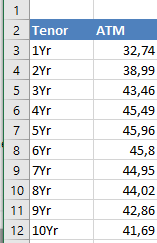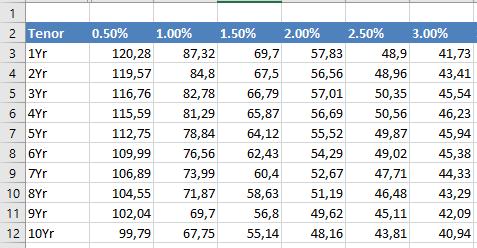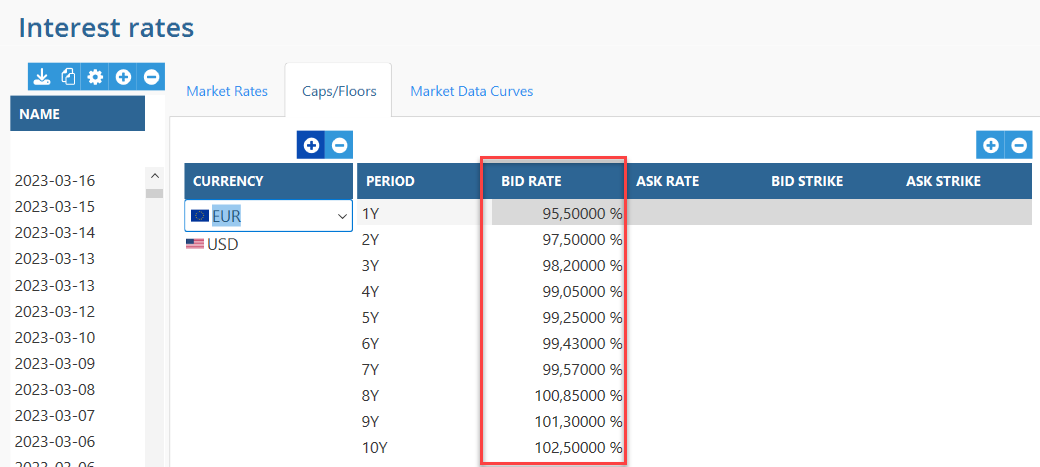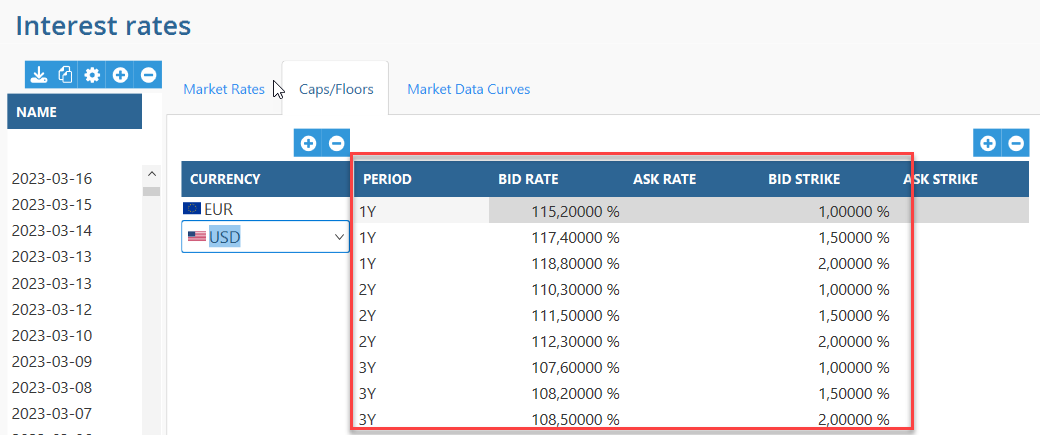# Market valuation of Caps/Floors

Created by Erik Åkerlund, Modified on Thu, 29 Jun 2023 at 04:26 PM by Teuvo Suoraniemi

To be able to market value caps and floors in TS we need market data in the system e.g. yield curves and volatilities based on different tenors. This is not included.

The market data for Caps/floors can, at the moment, be put in manually or imported via csv file.

In the system (TS) you can do the evaluation with a curve (the same strike at all tenors) or a surface (different strikes at the same tenor).

In a report the following columns can be used for Caps/Floors

Intrinsic value: The payoff of the Cap/Floor with today’s interest rates. Is equal to the market value at the exercise date.

It refers to the difference between the strike price of the option and the current price of the underlying asset.

Time Value: Time value refers to the portion of an option's value that is attributable to the amount of the time remaining until expiration of the option contract.

The residual of Market value - Intrinsic value. Is zero at the exercise date.

Market value Clean: Is calculated from current market data and excluding accrued interest.

Our calculation is based on Black-Scholes model. Requires Blacks (Log normal) volatilities.  Different valuation models may lead to different market valuations.

To handle valuation with negative interest rate in TS, TS apply shift to create the forward curve. The lowest quote will decide how much the curve will shift.

Mandatory fields in the file to import market date from file is:

Date; Name;Currency;Period;BidRate;BidStrike

Note decimal separator needs to be a dot (.)

Below is an example file

The file does not need to include strike rates if valuation shall be done with ATM, normal volatility where no strike rates are considered. If strike rates are imported anyway, then these strike rates will not be considered in the valuation because it is only one strike rate/volatility per tenor. If both a bid and ask rate shall be used fill in for both. If only Bid rates are filled in the Ask rates will be the same as the Bid rates if Mid rates are selected in the reports.

If different volatilities for different strike rates shall be considered for a more accurate market valuation Bid and/or Ask strikes shall be included.

In the example below, volatilities are for Normal volatility (ATM) where no strike rates are considered (a more simple market valuation). Strike rates shall not be filled in the Caps/floor tab in Interest rates.A more accurate market valuation is to use a "Surface" with volatilities per Strike rate. The tenors are the same as above. Then Strike rates need to be set up in the Interest rates table under Caps/FloorsExample below shows volatilities without strikes imported. If only Bid rates are filled in the Ask rates will be the same as the Bid rates.In the below example for USD volatility the volatilities for surface with strikes 1%, 1,5% and 2% are imported. If surface shall be used the strikes on the registered caps/floors in TS shall be within the intervals of strike rates.